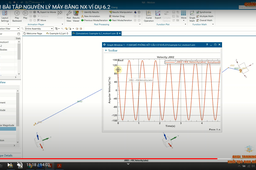Example 6.2. Locate all the instantaneous centres of the slider crank mechanism as shown in Fig. 6.12. The lengths of crank OB and connecting rod AB are 100 mm and 400 mm respectively. If the crank rotates clockwise with an angular velocity of 10 rad/s, fExample 6.2. Locate all the instantaneous centres of the slider crank mechanism as shown in Fig. 6.12. The lengths of crank OB and connecting rod AB are 100 mm and 400 mm respectively. If the crank rotates clockwise with an angular velocity of 10 rad/s, find:
1. Velocity of the slider A,
2. Angular velocity of the connecting rod AB

1. Step 1:

Example 6.2. Locate all the instantaneous centres of the slider crank mechanism as shown in Fig. 6.12. The lengths of crank OB and connecting rod AB are 100 mm and 400 mm respectively. If the crank rotates clockwise with an angular velocity of 10 rad/s, find:

1. Velocity of the slider A,

2. Angular velocity of the connecting rod AB